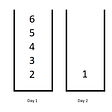# Solution to Leetcode’s Maximum Subarray

1. Have a global variable currentSum;
2. Have a global variable max that will also be the return value;
3. loop through the array, check if the current element (nums[i]) is greater than the current sum and current element put together (i.e currentSum + nums[i]);
4. if it is greater, reassign the currentSum variable to be the current element, nums[i];
5. if it is not, reassign the currentSum variable to be the summation of both the existing current sum and the current array element (currentSum = currentSum + nums[i])
6. finally, check if the currentSum is greater than the current maximum. if it is, reassign the current max to be equal to currentSum.
7. Then return max.
`public static int maxSubArray(int[] nums) {    int currentSum = nums;    int max = nums;    for (int i = 1; i < nums.length; i++) {        if (nums[i] > (currentSum + nums[i]))            currentSum = nums[i];        else            currentSum = currentSum + nums[i];        if (currentSum > max) {            max = currentSum;        }    }    return max;}`
`public static int maxSubArray(int[] nums) {    int currentSum = nums;    int max = nums;    for (int i = 1; i < nums.length; i++) {        //cleaner and simple        currentSum = Math.max(nums[i], currentSum + nums[i]);        max = Math.max(currentSum, max);    }    return max;}`
1. Don’t always been in the box of a particular algorithm you thought would work, just the way i thought Sliding window would work.
2. Using library functions where and when needed.

--

--

--

## More from Chunks

Love podcasts or audiobooks? Learn on the go with our new app.

## Data Exfiltration in Snowflake…## Research on how Kubernetes is used in Industries and what all use cases are solved by Kubernetes?## Point and click in Unity part 2## Notes on using Microsoft Graph SDK to manage users in an Azure AD B2C tenant.## Repelling A Ransomware Attack: Jon Toor of Cloudian On The 5 Things You Need To Do To Protect…## Introducing Deri Protocol Testnet## Word Pattern## LeetCode — Minimum Difficulty of a Job Schedule